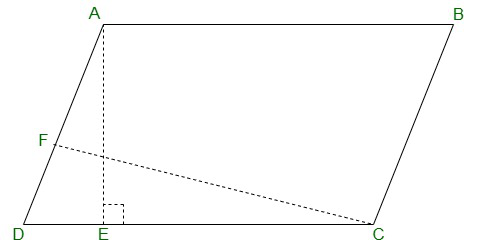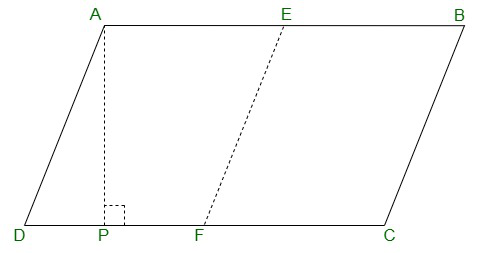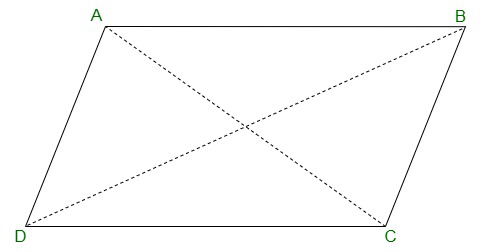Related Articles
Class 9 RD Sharma Solutions – Chapter 15 Areas of Parallelograms and Triangles- Exercise 15.2
• Last Updated : 28 Dec, 2020

### Question 1: If figure, ABCD is a parallelogram, AE ⊥ DC and CF ⊥ AD. If AB = 16 cm, AE = 8 cm and CF = 10 cm, find AD.Solution:

In parallelogram ABCD,
AB = CD = 16 cm {Since, opposite sides of a parallelogram are equal}
AE = 8 cm and CF = 10 cm
As we know,
Area of parallelogram = Base × Corresponding altitude
Area of parallelogram ABCD = CD × AE = AD × CF
16 × 18 = AD × 10
AD = 12.8
Thus, the length of AD = 12.8 cm

### Question 2: In Q.No. 1, if AD = 6 cm, CF = 10 cm and AE = 8 cm, find AB.Solution:

In parallelogram ABCD,
AE = 8 cm, CF = 10 cm and AD = 6 cm
As we know, Area of parallelogram = Base x Corresponding altitude
Area of parallelogram ABCD = AD × CF = CD × AE
6 × 10 = CD × 8
CD =  6 × 10  60
8            8
CD = 7.5 cm = AB {Since, opposite sides of a parallelogram are equal}
Thus, the length of AB = 7.5 cm

### Question 3: Let ABCD be a parallelogram of area 124 cm2. If E and F are the mid-points of sides AB and CD respectively, then find the area of parallelogram AEFD.

Solution:ABCD be a parallelogram.
Given:
Area of parallelogram = 124 cm2
Construct AP perpendicular to DC
Now,
Area of parallelogram EBCF = FC x AP
and
Area of parallelogram AFED = DF x AP
Here F is the mid-point of DC,
so DF = FC
Hence,
Area of parallelogram AEFD = Area of parallelogram EBCF =  (Area of parallelogram ABCD)
2
⇒  124
2
⇒ 62
Area of parallelogram AEFD is 62 cm2.

### Question 4: If ABCD is a parallelogram, then prove thatar(Δ ABD) = ar(Δ BCD) = ar(Δ ABC)=ar(Δ ACD) =  1  ar(||gm ABCD)                                                                                     2

Solution:Given:
ABCD is a parallelogram.

To prove : ar(Δ ABD) = ar(Δ BCD) = ar(Δ ABC)=ar(Δ ACD) =  ar(||gm ABCD)
2
Proof:
When we join the diagonal of parallelogram, it divides it into two equilaterals.
Since, AC is the diagonal.

Step 1: Area (ΔABC) = Area (ΔACD) =  (Area of ||gm ABCD)
2
Since, BD is the diagonal
Step 2: Area (ΔABD) = Area (ΔBCD) =  ( Area of ||gm ABCD)
Now,
From the above steps
Area (ΔABC) = Area (ΔACD) = Area (ΔABD) = Area (ΔBCD) =  (Area of ||gm ABCD)
2
Hence Proved.

Attention reader! Don’t stop learning now. Get hold of all the important DSA concepts with the DSA Self Paced Course at a student-friendly price and become industry ready.

My Personal Notes arrow_drop_up